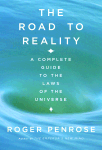## Tuesday, April 26, 2005

### "The Road to Reality" by Roger PenroseI've been dipping into Roger Penrose's new book, which presents an introduction (and beyond) to mathematical physics beginning with very elementary math - fractions!
Complex numbers are basic to understanding mathematical physics, especially quantum mechanics, moreover they're a useful and elegant tool throughout mathematics and physics. In particular there's quite a bit in the book about complex analysis. Basic texts and references for General Relativity and Quantum Theory (I myself own stacks of them) don't usually require much, if any, complex analysis - though it is certainly beautiful math and there are certain important applications in physics and elsewhere. That's an example of Penrose's idiosyncratic point of view, which sometimes I find a bit obscure but in other cases interesting, refreshing and provocative.

Here's a link to Martin Gardner's review in The New Criterion and to the review in the New York Times

In the beginning of the book Penrose presents a nice proof that the square root of two is an irrational number. This is hardly news, it dates to the time of Pythagoras, when it was supposedly considered so scandalous it was kept as a deep, dark secret. Probably this proof is well-know to everyone but me, but I had only remembered a proof involving prime decompositions and this one is more direct.

Assume there are positive integers p and q such that
(p/q)^2 = 2
so p^2 = 2q^2 and p must be even (because the square of an odd integer is also odd, but the square of p is even)
and hence p=2r for some positive integer r.
p^2 = (2r)^2 = 4r^2
2r^2 = q^2
So q is even as well, and q=2s for some positive integer s and furthermore p=4s.
2r^2 = 4s^2 and now s must be even, s=2t and p=8t.
This process can be repeated indefinitely, so p ends up having an infinite number of factors of two and hence p can't be a finite integer.
So the square root of two cannot be expressed as the ratio of two positive integers and hence is irrational.